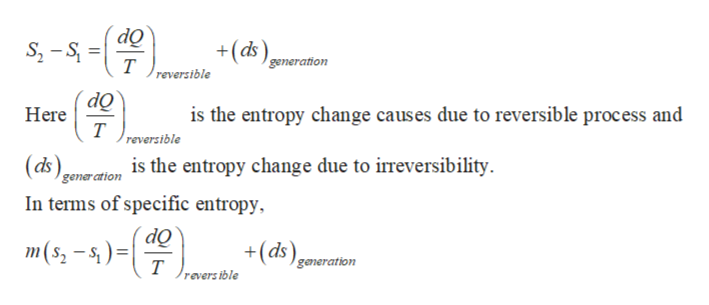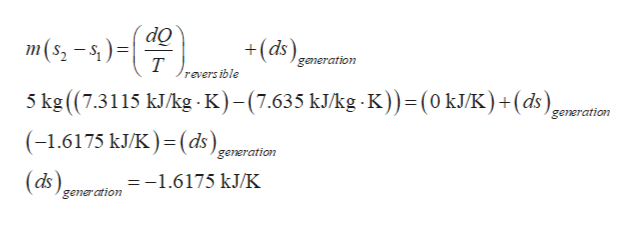# Five Kg of water contained in a piston-cylinder assembly expands from an initial state where T1 = 400o C, P1 = 700 KPa to a final state where T2 =200o C, P2 = 300 KPa, with no significant effects of kinetic and potential energy. The accompanying provides additional data at the two states. It is claimed that the water undergoes an adiabatic process between these states, while developing work. Evaluate this claimSTATE 1                                         STATE 2T1 = 400o C                                     T2 = 200O CP1 =700KPa = 7bar                        P2 =300KPa = 3 barv1= .4397 m3/kg                           v2=.7160 m3/kgu1 =2960.9 kj/kg                          u2=2650.7 kj/kgh1 = 3268.7 kj/kg                         h2=2865.5 kj/kgs1=7.6350 kj/kg * K                      s2=7.3115 lj/kg

Question
12 views

Five Kg of water contained in a piston-cylinder assembly expands from an initial state where T1 = 400o C, P1 = 700 KPa to a final state where T2 =200o C, P2 = 300 KPa, with no significant effects of kinetic and potential energy. The accompanying provides additional data at the two states. It is claimed that the water undergoes an adiabatic process between these states, while developing work. Evaluate this claim

STATE 1                                         STATE 2

T1 = 400o C                                     T2 = 200O C

P1 =700KPa = 7bar                        P2 =300KPa = 3 bar

v1= .4397 m3/kg                           v2=.7160 m3/kg

u1 =2960.9 kj/kg                          u2=2650.7 kj/kg

h1 = 3268.7 kj/kg                         h2=2865.5 kj/kg

s1=7.6350 kj/kg * K                      s2=7.3115 lj/kg

check_circle

Step 1

Consider the expression of the entropy change for the system,help_outlineImage TranscriptioncloseOps-s generation т reversible do Here т is the entropy change causes due to reversible process and reversible is the entropy change due to irreversibility as generation In terms of specific entropy m(s -s)= т +(ds generation revers ible fullscreen
Step 2

Consider the value provided in the problem, s1=7.635 Kj/kg K and s2=7.3115 Kj/kg K

For the checking the claim, assume the process is adiabatic. Thus, the hea...help_outlineImage TranscriptionclosedQ m(s, – s, ) =| +(ds) generation т revers ible 5 kg ((7.3115 kJ/kg - K)-(7.635 kJ/kg -K))=(0 kJ/K)+(ds), (-1.6175 kJ/K )=(ds)genæration generation (ds), =-1.6175 kJ/K gener ation fullscreen

### Want to see the full answer?

See Solution

#### Want to see this answer and more?

Solutions are written by subject experts who are available 24/7. Questions are typically answered within 1 hour.*

See Solution
*Response times may vary by subject and question.
Tagged in

### Mechanical Engineering